## Selina Concise Mathematics Class 10 ICSE Solutions Chapter 11 Geometric Progression Ex 11A

These Solutions are part of Selina Concise Mathematics Class 10 ICSE Solutions. Here we have given Selina Concise Mathematics Class 10 ICSE Solutions Chapter 11 Geometric Progression Ex 11A

Other Exercises

Question 1.
Find, which of the following sequence form a G.P. :
(i) 8, 24, 72, 216, ……
(ii) $$\\ \frac { 1 }{ 8 }$$,$$\\ \frac { 1 }{ 24 }$$,$$\\ \frac { 1 }{ 72 }$$,$$\\ \frac { 1 }{ 216 }$$
(iii) 9, 12, 16, 24,…..
Solution:
(i) 8, 24, 72, 216,……
Here, a = 8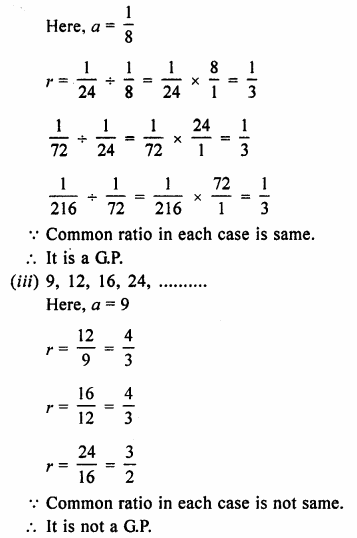Question 2.
Find the 9th term of the series :
1, 4, 16, 64,…….
Solution:
In G.P. 1, 4, 16, 64,….
Here first term (a) = 1
and common ratio (r) = $$\\ \frac { 4 }{ 1 }$$ = 4,
T9 = arn – 1 = 1 x 49 – 1 = 1 x 48 = 48
= 4 x 4 x 4 x 4 x 4 x 4 x 4 x 4
= 65536

Question 3.
Find the seventh term of the G.P. :
1 , √3, 3, 3√3…….
Solution:
G.P. is 1 , √3, 3, 3√3
Here first term (a) = 1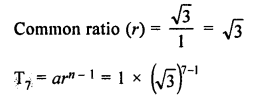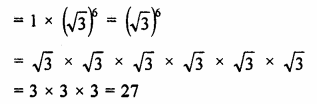Question 4.
Find the 8th term of the sequence :
$$\\ \frac { 3 }{ 4 }$$,$$1 \frac { 1 }{ 2 }$$,3……
Solution:
G.P. = $$\\ \frac { 3 }{ 4 }$$,$$1 \frac { 1 }{ 2 }$$,3…….
= $$\\ \frac { 3 }{ 4 }$$,$$\\ \frac { 3 }{ 2 }$$,3…….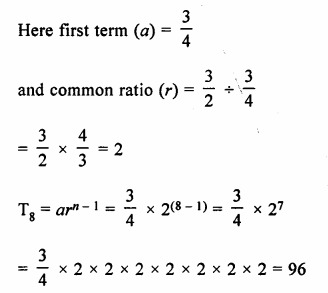Question 5.
Find the 10th term of the G.P. :
12, 4, $$1 \frac { 1 }{ 3 }$$,……
Solution:
G.P. = 12, 4, $$1 \frac { 1 }{ 3 }$$,……..
= 12, 4, $$\\ \frac { 4 }{ 3 }$$,…..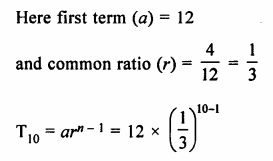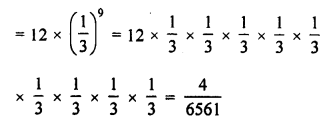Question 6.
Find the nth term of the series :
1, 2, 4, 8 …….
Solution:
1, 2, 4, 8,……
Here, a = 1,r = $$\\ \frac { 2 }{ 1 }$$ = 2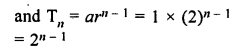Question 7.
Find the next three terms of the sequence :
√5, 5, 5√5…..
Solution:
√5, 5, 5√5……
Here a = √5 and r = $$\frac { 5 }{ \surd 5 }$$ = √5Question 8.
Find the sixth term of the series :
22, 23, 24,….
Solution:
22, 23, 24,……
Here, a = 22, r = 23 ÷ 22 = 23 – 2 = 21 = 2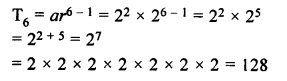Question 9.
Find the seventh term of the G.P. :
√3 + 1, 1, $$\frac { \surd 3-1 }{ 2 }$$,…….
Solution:
√3 + 1, 1, $$\frac { \surd 3-1 }{ 2 }$$,…….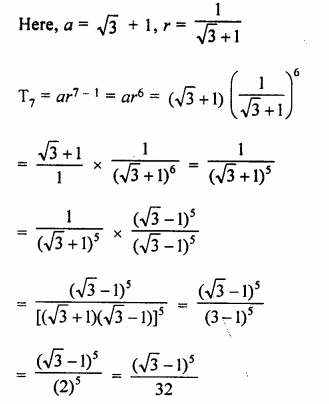Question 10.
Find the G.P. whose first term is 64 and next term is 32.
Solution:
First term of a G.P. (a) = 64
and second term (ar) = 32
G.P. will be 64, 32, 16, 8, 4, 2, 1,…….

Question 11.
Find the next three terms of the series:
$$\\ \frac { 2 }{ 27 }$$,$$\\ \frac { 2 }{ 9 }$$,$$\\ \frac { 2 }{ 3 }$$,…..
Solution:
G.P. is $$\\ \frac { 2 }{ 27 }$$,$$\\ \frac { 2 }{ 9 }$$,$$\\ \frac { 2 }{ 3 }$$,…..
a = $$\\ \frac { 2 }{ 27 }$$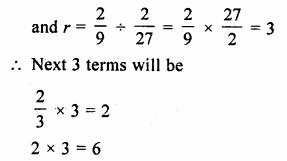Question 12.
Find the next two terms of the series
2 – 6 + 18 – 54…..
Solution:
G.P. is 2 – 6 + 18 – 54 +………
Here a = 2 and r = $$\\ \frac { -6 }{ 2 }$$ = – 3
Next two terms will be
– 54 x ( – 3) = + 162
162 x ( – 3) = – 486
Next two terms are 162 – 486

Hope given Selina Concise Mathematics Class 10 ICSE Solutions Chapter 11 Geometric Progression Ex 11A are helpful to complete your math homework.

If you have any doubts, please comment below. Learn Insta try to provide online math tutoring for you.